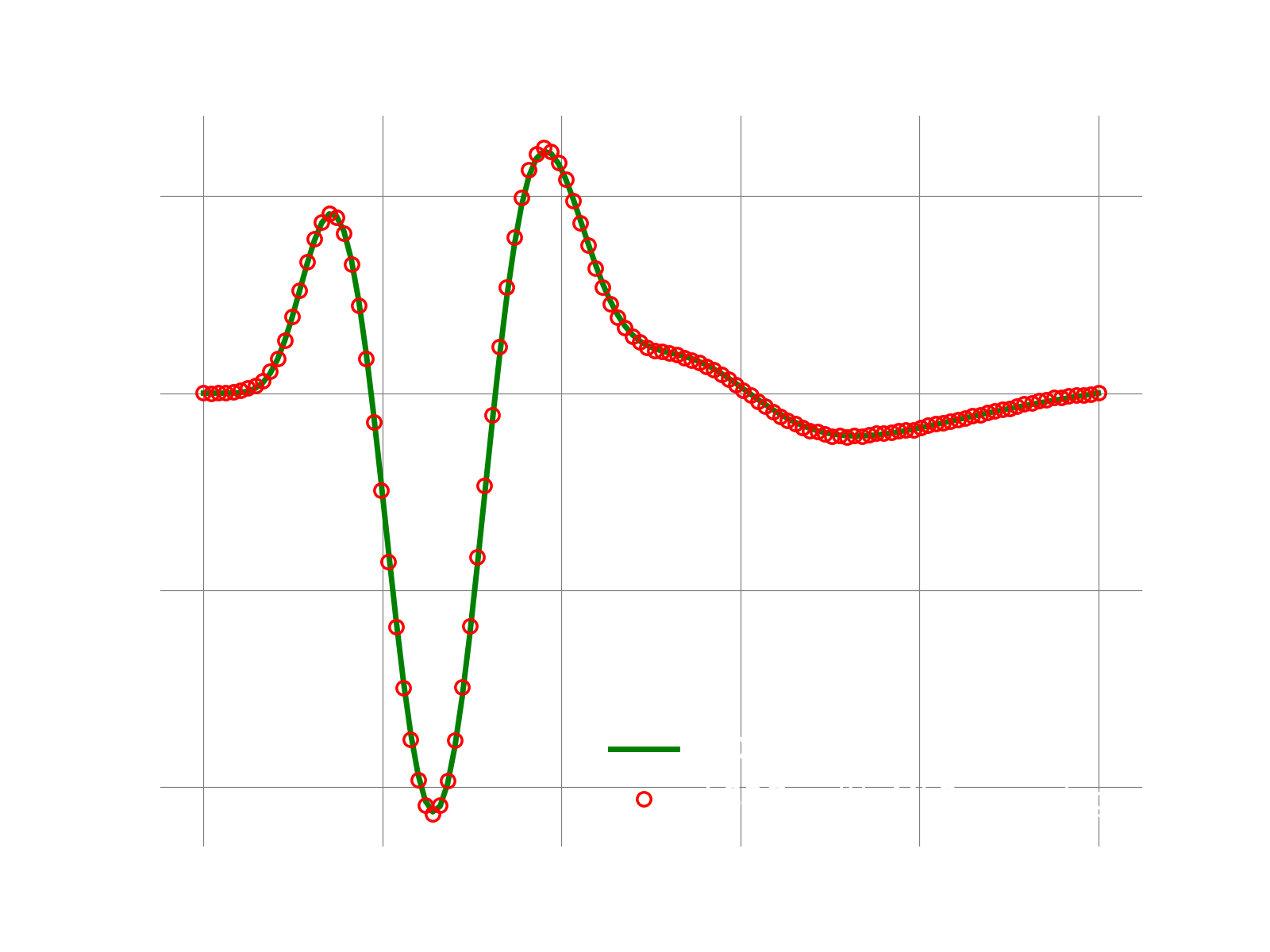# 1D adv-diff: LSPG with nonlinear manifold projection via MLP

## Overview

This demo solves the same problem as the one here, but instead of using POD modes, we show here how to use a nonlinear manifold computed approximated by a neural network. Specifically, we use a MLP with 2 hidden layers of sizes 64 and 200.

## Main function

The main function of the demo is the following:

```logger.initialize(logger.logto.terminal)
logger.setVerbosity([logger.loglevel.info])

# create fom object

# the final time to integrate to
finalTime = .05

#--- 1. FOM ---#
fomTimeStepSize  = 1e-5
fomNumberOfSteps = int(finalTime/fomTimeStepSize)
sampleEvery      = 200
[fomFinalState, snapshots] = doFom(fomObj, fomTimeStepSize, fomNumberOfSteps, sampleEvery)

#--- 2. train a nonlinear mapping using PyTorch ---#
# here we use 3 modes, change this to try different modes
myNonLinearMapper = trainMapping(snapshots, romSize=3, epochs=500)

#--- 3. LSPG ROM ---#
romTimeStepSize  = 3e-4
romNumberOfSteps = int(finalTime/romTimeStepSize)
approximatedState = runLspg(fomObj, romTimeStepSize, romNumberOfSteps, myNonLinearMapper)

# compute l2-error between fom and approximate state
fomNorm = linalg.norm(fomFinalState)
err = linalg.norm(fomFinalState-approximatedState)
print("Final state relative l2 error: {}".format(err/fomNorm))

logger.finalize()```

### 1. Run FOM and collect snapshots

This step is the same as described here,

### 2. Setup and train the nonlinear mapper

It is important to note that while the mapper class below has the API required by pressio4py, it can encapsulate any arbitrary mapping function. In this case we show how to create a MLP-based representation in PyTorch, but one can use any other types of mapping and any other library (e.g., Tensorflow, keras). All of the PyTorch-specific code is encapsulated here. If you prefer Tensorflow/keras, an equivalent implementation is here.

The autoencoder is defined by

```class myAutoencoder(torch.nn.Module):
def __init__(self, fomSize, romSize=10):
super(myAutoencoder, self).__init__()
self.encoder = myEncoder(fomSize, romSize)
self.decoder = myDecoder(fomSize, romSize)

def forward(self, x):
code = self.encoder(x)
x = self.decoder(code)
return x, code

def train(self, dataloader, optimizer, n_epochs, loss=torch.nn.MSELoss()):
scheduler = torch.optim.lr_scheduler.ReduceLROnPlateau(optimizer, factor=0.8, min_lr=1e-6)
for epoch in range(n_epochs):
total_train_loss = 0.0
output, latent = self.forward(data)
loss_size = loss(output, label)
loss_size.backward()
optimizer.step()
total_train_loss += loss_size.item()
scheduler.step(total_train_loss)

class myEncoder(torch.nn.Module):
def __init__(self, fomSize, romSize):
super(myEncoder, self).__init__()
self.fc1 = torch.nn.Linear(fomSize, 200)
self.fc2 = torch.nn.Linear(200, 64)
self.fc3 = torch.nn.Linear(64, romSize)

def forward(self, x):
x = self.fc1(x)
x = F.elu(x)
x = self.fc2(x)
x = F.elu(x)
x = self.fc3(x)
x = F.elu(x)
return x

class myDecoder(torch.nn.Module):
def __init__(self, fomSize, romSize):
super(myDecoder, self).__init__()
self.romSize_ = romSize
self.fomSize_ = fomSize
self.fc1 = torch.nn.Linear(romSize, 64)
self.fc2 = torch.nn.Linear(64, 200)
self.fc3 = torch.nn.Linear(200, fomSize)

def forward(self, x):
x = self.fc1(x)
x = F.elu(x)
x = self.fc2(x)
x = F.elu(x)
x = self.fc3(x)
return x```

and is created/trained using

```def trainMapping(snapshots, romSize, epochs, enable_restart=False):
fomSize = snapshots.shape
model = myAutoencoder(fomSize, romSize)

if enable_restart:
if pathlib.Path('TrainingCheckpoint.tar').is_file():

samples = torch.utils.data.TensorDataset(torch.Tensor(snapshots.T), torch.Tensor(snapshots.T))

if enable_restart:
torch.save({
'model_state_dict': model.state_dict(),
'optimizer_state_dict': optimizer.state_dict()
},
'TrainingCheckpoint.tar')

return MyMapper(model.decoder, model.encoder)```

This is all wrapped in a mapper class which conforms to the API required by Pressio

```class MyMapper:
def __init__(self, decoderObj, encoderObj):
self.decoder_ = decoderObj
self.encoder_ = encoderObj

self.numModes_ = decoderObj.romSize_
fomSize = decoderObj.fomSize_
self.fomState0 = np.zeros(fomSize)
self.fomState1 = np.zeros(fomSize)
# attention: the jacobian of the mapping must be column-major oder
# so that pressio can view it without deep copying it, this enables
# to keep only one jacobian object around and to call the update
# method below correctly
self.jacobian_ = np.zeros((fomSize,self.numModes_), order='F')

def jacobian(self): return self.jacobian_

def applyMapping(self, romState, fomState):
fomState[:] = self.decoder_(torch.Tensor(romState)).detach().numpy()

def applyInverseMapping(self, fomState):
romState = np.zeros(self.numModes_)
romState[:] = self.encoder_(torch.Tensor(fomState)).detach()[:]
return romState

def updateJacobian(self, romState):
self.updateJacobianFD(romState)

def updateJacobianFD(self, romState):
# finite difference to approximate jacobian of the mapping
romStateLocal = romState.copy()
self.applyMapping(romStateLocal,self.fomState0)
eps = 0.001
for i in range(self.numModes_):
romStateLocal[i] += eps
self.applyMapping(romStateLocal, self.fomState1)
self.jacobian_[:,i] = (self.fomState1 - self.fomState0) / eps
romStateLocal[i] -= eps

def updateJacobianExact(self, romState):
# use pytorch autodifferentiation to compute jacobian of the mapping
# slower than finite difference currently
self.jacobian_[:,:] = J.detach()[:,:]```

### 3. Construct and run LSPG

```def runLspg(fomObj, dt, nsteps, customMapper):
# this is an auxiliary class that can be passed to solve
# LSPG to monitor the rom state.
class RomStateObserver:
def __call__(self, timeStep, time, state): pass

# this linear solver is used at each gauss-newton iteration
class MyLinSolver:
def solve(self, A,b,x):
lumat, piv, info = linalg.lapack.dgetrf(A, overwrite_a=True)
x[:], info = linalg.lapack.dgetrs(lumat, piv, b, 0, 0)

#----------------------------------------
# create a custom decoder using the mapper passed as argument
customDecoder = rom.Decoder(customMapper, "MyMapper")

# fom reference state: here it is zero
fomReferenceState = np.zeros(fomObj.nGrid)

# create ROM state by projecting the fom initial condition
fomInitialState = fomObj.u0.copy()
romState = customMapper.applyInverseMapping(fomInitialState)

# create LSPG problem
scheme = ode.stepscheme.BDF1
problem = rom.lspg.unsteady.DefaultProblem(scheme, fomObj, customDecoder, romState, fomReferenceState)

# create the Gauss-Newton solver
nonLinSolver = solvers.create_gauss_newton(problem, romState, MyLinSolver())
# set tolerance and convergence criteria
nlsTol, nlsMaxIt = 1e-7, 10
nonLinSolver.setMaxIterations(nlsMaxIt)
nonLinSolver.setStoppingCriterion(solvers.stop.WhenCorrectionAbsoluteNormBelowTolerance)

# create object to monitor the romState at every iteration
myObs = RomStateObserver()
# solve problem
ode.advance_n_steps_and_observe(problem, romState, 0., dt, nsteps, myObs, nonLinSolver)

# after we are done, use the reconstructor object to reconstruct the fom state
# get the reconstructor object: this allows to map romState to fomState
fomRecon = problem.fomStateReconstructor()
return fomRecon(romState)```

## Results

If everything works fine, the following plot shows the result.